Checkout JEE MAINS 2022 Question Paper Analysis : Checkout JEE MAINS 2022 Question Paper Analysis :

# Ohm's Law

Ohm’s law states the relationship between electric current and potential difference. The current that flows through most conductors is directly proportional to the voltage applied to it. Georg Simon Ohm, a German physicist was the first to verify Ohm’s law experimentally.

## Ohm’s Law Explanation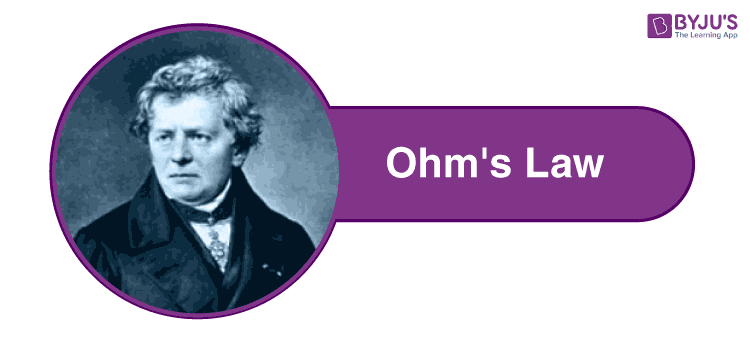One of the most basic and important laws of electric circuits is Ohm’s law.

Ohm’s law states that the voltage across a conductor is directly proportional to the current flowing through it, provided all physical conditions and temperatures remain constant.

Mathematically, this current-voltage relationship is written as,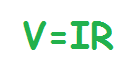In the equation, the constant of proportionality, R, is called Resistance and has units of ohms, with the symbol Ω.

The same formula can be rewritten in order to calculate the current and resistance respectively as follows: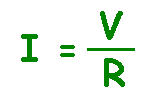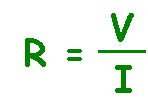Ohm’s law only holds true if the provided temperature and the other physical factors remain constant. In certain components, increasing the current raises the temperature. An example of this is the filament of a light bulb, in which the temperature rises as the current is increased. In this case, Ohm’s law cannot be applied. The lightbulb filament violates Ohm’s Law.

 Ohm’s Law Statement: Ohm’s law states that the voltage across a conductor is directly proportional to the current flowing through it, provided all physical conditions and temperature, remain constant. Ohm’s Law Equation: V = IR, where V is the voltage across the conductor, I is the current flowing through the conductor and R is the resistance provided by the conductor to the flow of current.

### Relationship Between Voltage, Current and Resistance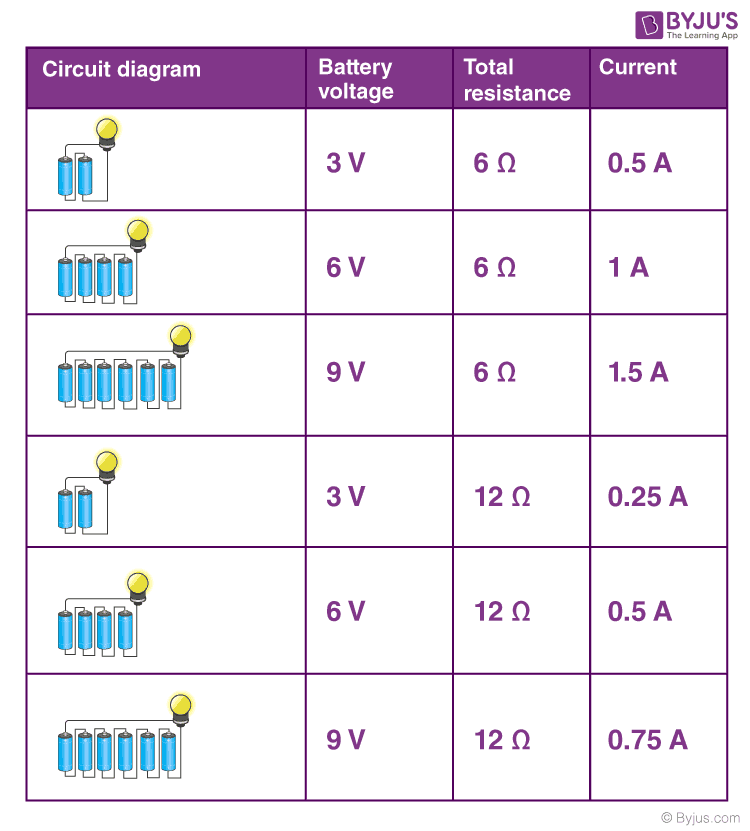Analyzing rows 1, 2 and 3, we come to understand that doubling and tripling the voltage leads to doubling and a tripling of the current in the circuit. Likewise, when we compare rows 1 and 4 and rows 2 and 5, we come to understand that doubling the total resistance serves to halve the current in the circuit.

### Water Pipe Analogy for Ohm’s Law

Ohm’s Law describes the current flow through a resistance when different electric potentials (voltage) are applied at each end of the resistance. Since we can’t see electrons, the water-pipe analogy helps us understand the electric circuits better. Water flowing through pipes is a good mechanical system that is analogous to an electrical circuit.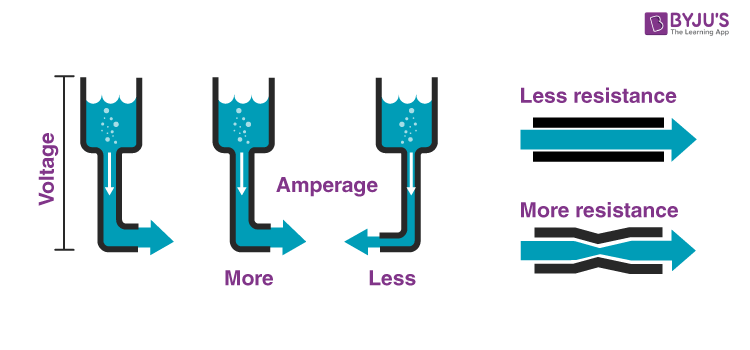Here, the voltage is analogous to water pressure, the current is the amount of water flowing through the pipe, and the resistance is the size of the pipe. More water will flow through the pipe (current) when more pressure is applied (voltage) and the bigger the pipe (lower the resistance).

The video below shows the physical demonstration of the Waterpipe analogy and explains to you the factors that affect the flow of current.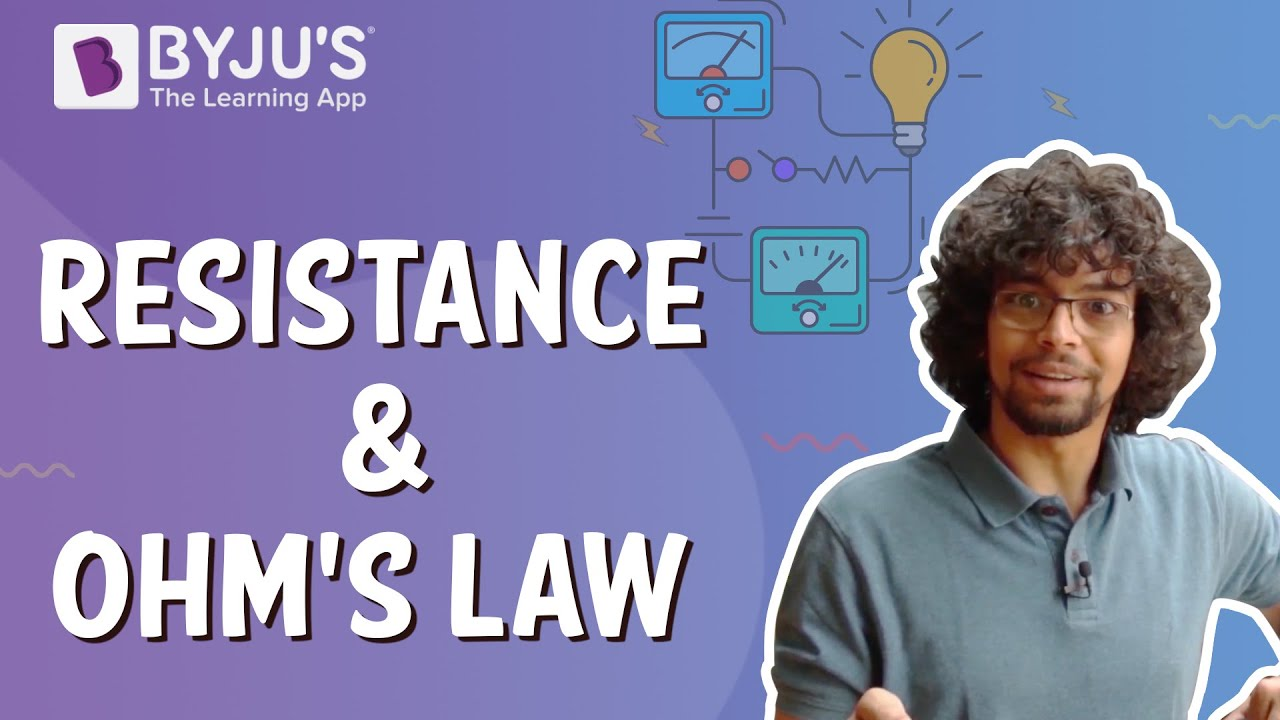## Experimental Verification of Ohm’s Law

Ohm’s Law can be easily verified by the following experiment:

Apparatus Required:

• Resistor
• Ammeter
• Voltmeter
• Battery
• Plug Key
• Rheostat

Circuit Diagram: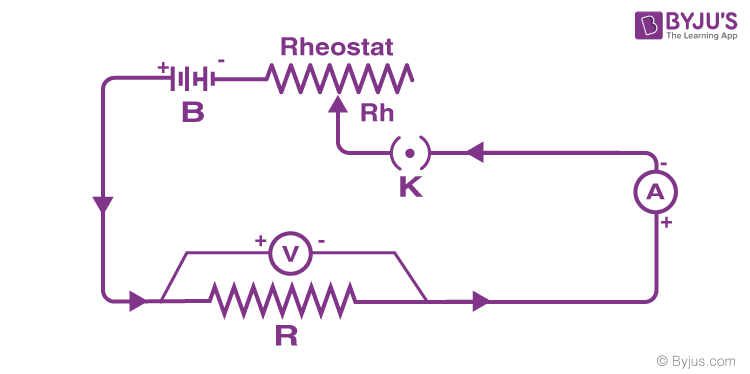Procedure:

1. Initially, the key K is closed and the rheostat is adjusted to get the minimum reading in ammeter A and voltmeter V.
2. The current in the circuit is increased gradually by moving the sliding terminal of the rheostat. During the process, the current flowing in the circuit and the corresponding value of potential difference across the resistance wire R are recorded.
3. This way different sets of values of voltage and current are obtained.
4. For each set of values of V and I, the ratio of V/I is calculated.
5. When you calculate the ratio V/I for each case, you will come to notice that it is almost the same. So V/I = R, which is a constant.
6. Plot a graph of the current against the potential difference, it will be a straight line. This shows that the current is proportional to the potential difference.

## Ohm’s Law Magic Triangle

You can make use of Ohm’s law magic triangle to remember the different equations for Ohm’s law used to solve for different variables(V, I, R).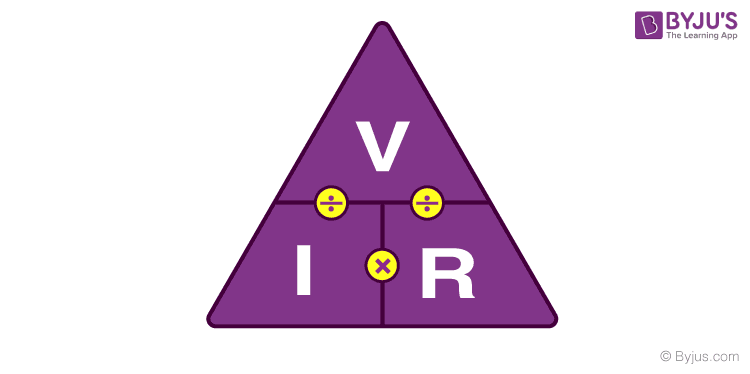If the value of voltage is asked and the values of the current and resistance are given, then to calculate voltage simply cover V at the top. So, we are left with the I and R or I × R. So, the equation for Voltage is Current multiplied by Resistance. Examples of how the magic triangle is employed to determine the voltage using Ohm’s law are given below.

### Ohm’s Law Solved Problems

Example 1: If the resistance of an electric iron is 50 Ω and a current of 3.2 A flows through the resistance. Find the voltage between two points.

Solution:

If we are asked to calculate the value of voltage with the value of current and resistance, then cover V in the triangle. Now, we are left with I and R or more precisely I × R.

Therefore, we use the following formula to calculate the value of V:

V = I × R

Substituting the values in the equation, we get

V = 3.2 A × 50 Ω = 160 V

 V = 160V

Example 2: An EMF source of 8.0 V is connected to a purely resistive electrical appliance (a light bulb). An electric current of 2.0 A flows through it. Consider the conducting wires to be resistance-free. Calculate the resistance offered by the electrical appliance.

Solution:

When we are asked to determine the value of resistance when the values of voltage and current are given, we cover R in the triangle. This leaves us with only V and I, more precisely V ÷ I.

Substituting the values in the equation, we get

R = V ÷ I

R = 8 V ÷ 2 A = 4 Ω

 R = 4 Ω

## Calculating Electrical Power Using Ohm’s Law

The rate at which energy is converted from the electrical energy of the moving charges to some other form of energy like mechanical energy, heat energy, energy stored in magnetic fields or electric fields, is known as electric power. The unit of power is the watt. The electrical power can be calculated using Ohm’s law and by substituting the values of voltage, current and resistance.

### Formula to find power

When the values for voltage and current are given,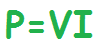When the values for voltage and resistance are given,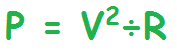When the values for current and resistance are given,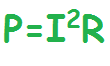### What is a Power Triangle?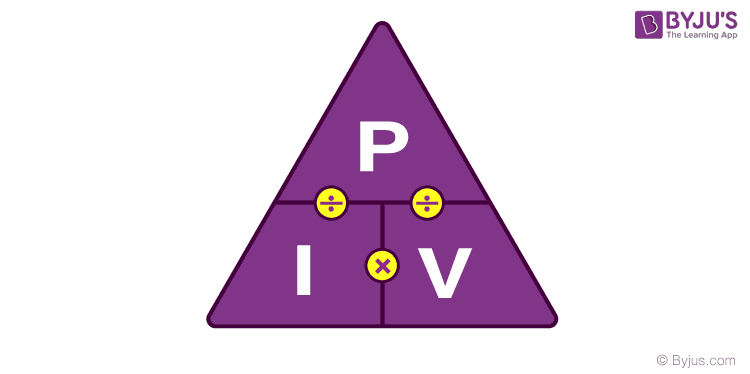The power triangle can be employed to determine the value of electric power, voltage and current when the values of the other two parameters are given to us. In the power triangle, the power (P) is on the top and current (I) and voltage (V) are at the bottom.

When the values of current and voltage are given, the formula for finding power is,When the values of power and voltage are given, the formula for finding current is,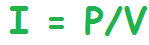When the values of power and current are given, the formula for finding voltage is,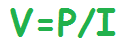## Ohm’s Law Pie Chart

To better understand the relationship between various parameters, we can take all the equations used to find the voltage, current, resistance and power, and condense them into a simple Ohm’s Law pie chart as shown below.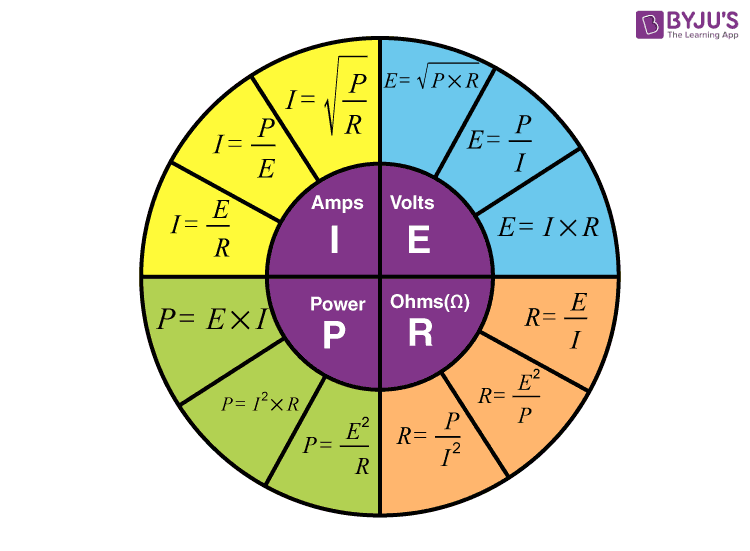## Ohm’s Law Matrix Table

Like Ohm’s Law Pie Chart shown above, we can condense the individual Ohm’s Law equations into a simple matrix table as shown below for easy reference when calculating an unknown value.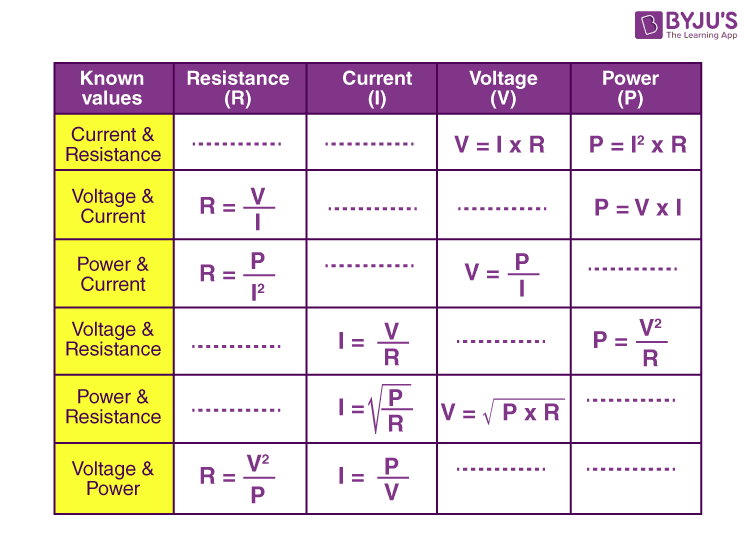## Ohm’s Law Applications

The main applications of Ohm’s law are:

• To determine the voltage, resistance or current of an electric circuit.
• Ohm’s law maintains the desired voltage drop across the electronic components.
• Ohm’s law is also used in DC ammeter and other DC shunts to divert the current.

### Limitations of Ohm’s Law

Following are the limitations of Ohm’s law:

• Ohm’s law is not applicable for unilateral electrical elements like diodes and transistors as they allow the current to flow through in one direction only.
• For non-linear electrical elements with parameters like capacitance, resistance etc the ratio ofvoltage and current won’t be constant with respect to time making it difficult to use Ohm’s law.

## Frequently Asked Questions – FAQs

### What does Ohm’s law state?

Ohm’s law states that the current through a conductor between two points is directly proportional to the voltage across the two points.

### What can Ohm’s law be used for?

Ohm’s law is used to validate the static values of circuit components such as current levels, voltage supplies, and voltage drops.

### Is Ohm’s law Universal?

No. Ohm’s law is not a universal law. This is because Ohm’s law is only applicable to ohmic conductors such as iron and copper but is not applicable to non-ohmic conductors such as semiconductors.

### Why is Ohm’s law not applicable to semiconductors?

Ohm’s law doesn’t apply to semiconducting devices because they are nonlinear devices. This means that the ratio of voltage to current doesn’t remain constant for variations in voltage.

### When does Ohm’s law fail?

Ohm’s law fails to explain the behaviour of semiconductors and unilateral devices such as diodes. Ohm’s law may not give the desired results if the physical conditions such as temperature or pressure are not kept constant.

Stay tuned to BYJU’S and Fall in Love with Learning!

Test your Knowledge on Ohms Law

1. Venkatesh

Nice app to a students

2. abdullah

thanks sir

3. Tayerera kudzai

Nice app for us

4. Very good

nice thank u sir

6. Mishu Mazumder Sourov

Very useful study material

Good explanation!

8. These are good material

Like
Very thanks 💐 byju ‘s team